## GATE 2005

 Question 1

What does the following C-statement declare?

`    int ( * f) (int * ) ; `
 A A function that takes an integer pointer as argument and returns an integer. B A function that takes an integer as argument and returns an integer pointer. C A pointer to a function that takes an integer pointer as argument and returns an integer. D A function that takes an integer pointer as argument and returns a function pointer.
Programming       C-Programming
Question 1 Explanation:
int ( * f) (int * )
→ A pointer to a function which takes integer as a pointer and return an integer value.
 Question 2

An Abstract Data Type (ADT) is:

 A Same as an abstract class B A data type that cannot be instantiated C A data type type for which only the operations defined on it can be used, but none else D All of the above
Data-Structures       Abstract-Data-Type
Question 2 Explanation:
An Abstract datatype is a type for objects whose behaviour is defined by set of values and set of operations.
 Question 3

A common property of logic programming languages and functional languages is:

 A both are procedural languages B both are based on λ-calculus C both are declarative D both use Horn-clauses
Programming       Programming-Paradigm
Question 3 Explanation:
Functional and logic programming languages are also called declarative languages; programs in these languages are said to describe (declaratively) what to do and not (operationally) how to do it.
 Question 4

Which one of the following are essential features of an object-oriented programming language?

(i) Abstraction and encapsulation
(ii) Strictly-typedness
(iii) Type-safe property coupled with sub-type rule
(iv) Polymorphism in the presence of inheritance
 A (i) and (ii) only B (i) and (iv) only C (i), (ii) and (iv) only D (i), (iii) and (iv) only
Programming       Programming-Paradigm
Question 4 Explanation:
Abstraction, encapsulation and inheritance are three main features of OOP language.
 Question 5

A program P reads in 500 integers in the range [0, 100] representing the scores of 500 students. It then prints the frequency of each score above 50. What would be the best way for P to store the frequencies?

 A An array of 50 numbers B An array of 100 numbers C An array of 500 numbers D A dynamically allocated array of 550 numbers
Data-Structures       Arrays
Question 5 Explanation:
→ Here we are storing values above 50 and we are ignoring the scores which is less than 50.
→ Then using array of 50 numbers is the best way to store the frequencies.
 Question 6

An undirected graph C has n nodes. Its adjacency matrix is given by an n × n square matrix whose (i) diagonal elements are 0's and (ii) non-diagonal elements are l's. Which one of the following is TRUE?

 A Graph G has no minimum spanning tree (MST) B Graph G has a unique MST of cost n-1 C Graph G has multiple distinct MSTs, each of cost n-1 D Graph G has multiple spanning trees of different costs
Data-Structures       Graphs
Question 6 Explanation:
From given data, we can say that the given graph is complete graph with all edge weights 1. Hence weight of MST is n-1.
Since the weights of every edge is 1 so there are different MST's are possible with same cost, i.e., (n-1).
 Question 7

The time complexity of computing the transitive closure of a binary relation on a set of n elements is known to be:

 A O(n) B O(n log n) C O(n3/2) D O(n3)
Algorithms        Time-Complexity
Question 7 Explanation:
First of all, In mathematics the transitive closure of a binary relation R on a set X is defined as the smallest relation on X that contains R and is transitive. More formally, the transitive closure of a binary relation R on a set X is the transitive relation R+ on set X such that R+ contains R and R+ is minimal.
For example, if X is a set of airports and x R y means "there is a direct flight from airport x to airport y" (for x and y in X), then the transitive closure of R on X is the relation R+ such that x R+ y means "it is possible to fly from x to y in one or more flights". Informally, the transitive closure gives you the set of all places you can get to from any starting place.
To find the transitive closure of given relation, we can represent the given relation by the graph such that if x R y then there should be directed edge between x and y in a graph. For this graph, we have to apply modified Floyd_Warshall Algorithm to find the transitive closure of the graph.
The time complexity of this algorithm is O(V3) where V is the number of vertices in the given graph. You can take here V as the number of elements is set i.e., N. Therefore time complexity for the given question is O(n3).
 Question 8

Let A, B and C be non-empty sets and let X = (A - B) - C and Y = (A - C) - (B - C). Which one of the following is TRUE?

 A X = Y B X ⊂ Y C Y ⊂ X D None of these
Engineering-Mathematics       Sets-And Relation
Question 8 Explanation:
Consider, A = {1, 2, 3, 4, 5, 6}
B = {1, 3, 4, 5}
C = {2, 4, 5, 6}
X = (A - B) - C
X = {2, 6} - {2, 4, 5, 6}
= ∅
Y = (A - C) - (B - C)
= {1, 3} - { 1, 3}
= ∅
X = YX = (A - B) - C
= (1, 5) - (5, 7, 4, 3)
= (1)
Y = (A - C) - (B - C)
= (1, 4) - (2, 4)
= (1)
X = Y
 Question 9

The following is the Hasse diagram of the poset [{a,b,c,d,e},<]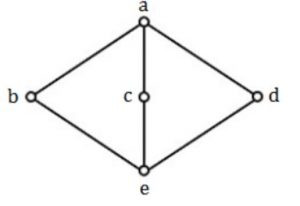The poset is

 A not a lattice B a lattice but not a distributive lattice C a distributive lattice but not a Boolean algebra D a Boolean algebra
Engineering-Mathematics        Sets-And-Relation
Question 9 Explanation:
It is lattice because both LUB and GLB exist for each pair of the vertex in above Hasse diagram.
But it is not distributed because for some element there are more than one complement. Moreover if it is not distributive then it can't be boolean.
 Question 10

Let G be a simple connected planar graph with 13 vertices and 19 edges. Then, the number of faces in the planar embedding of the graph is:

 A 6 B 8 C 9 D 13
Engineering-Mathematics       Graph-Theory
Question 10 Explanation:
No. of faces in a planar embedding of a graph is
F = E - V + 2 [From Euler's formula i.e., F + V - E = 2]
F = 19 - 13 +2
F = 8
 Question 11

Let G be a simple graph with 20 vertices and 100 edges. The size of the minimum vertex cover of G is 8. Then, the size of the maximum independent set of G is

 A 12 B 8 C Less than 8 D More than 12
Engineering-Mathematics       Graph-Theory
Question 11 Explanation:
No. of vertices = 20
Edges = 100
Minimum cover of vertex G is = 8
Maximum Independent set of G = No. of vertices - Minimum cover of vertex G
= 20 - 8
= 12
 Question 12

Let f(x) be the continuous probability density function of a random variable X. The probability that a < X ≤ b, is:

 A f(b - a) B f(b) - f(a) CDEngineering-Mathematics       Probability
Question 12 Explanation:
f(x) be the continuous probability density function of random variable X.
Then the probablity be area of the corresponding curve i.e.,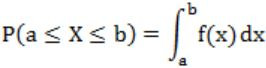Question 13

The set {1, 2, 4, 7, 8, 11, 13, 14} is a group under multiplication modulo 15. The inverses of 4 and 7 are respectively:

 A 3 and 13 B 2 and 11 C 4 and 13 D 8 and 14
Engineering-Mathematics       Sets-And Relation
Question 13 Explanation:
Let say,
Inverse of 4 = m; Inverse of 7 = n
(4×m)%15=1; (7*n)%15=1
Option A: m=3 n=13
12%15≠1 (✖️) 91%15=1 (✔️)
Option B: m=2 n=11
8%15≠1 (✖️) 11%15≠1 (✖️)
Option C: m=4 n=13
16%15=1(✔️) 91%15=1 (✔️)
Option D: m=8 n=14
120%15≠1(✖️) 98%15≠1(✖️)
 Question 14

The grammar A → AA | (A) | ε is not suitable for predictive-parsing because the grammar is:

 A ambiguous B left-recursive C right-recursive D an operator-grammar
Compiler-Design       Parsers
Question 14 Explanation:
The given grammar can be turned into a infinite parse tree. So it is ambiguous.
It have A → AA has left recursion.
 Question 15

Consider the following circuit.Which one of the following is TRUE?

 A f is independent of X B f is independent of Y C f is independent of Z D None of X, Y, Z is redundant
Digital-Logic-Design       Circuits-Output
Question 15 Explanation:
f(X,Y,Z) = ((XY’)’ (YZ))’
= ((X’+Y) YZ)’
= (X’YZ + YZ)’
= ((X’+1) YZ)’
= (YZ)’
 Question 16

The range of integers that can be represented by an n bit 2's complement number system is:

 A - 2n-1 to (2n-1 - 1) B - (2n-1 - 1) to (2n-1 - 1) C - 2n-1 to 2n-1 D - (2n-1 + 1) to (2n-1 - 1)
Digital-Logic-Design       Number-Systems
Question 16 Explanation:
The maximum (positive) n bit number is 011….1 (i.e., 0 followed by n-1 ones) which is equal to 2n-1 - 1.
The smallest (negative) n bit number is 100..0 (i.e., 1 followed by n-1 zeros) which is equal to - 2n-1.
1000...00
0111...11 <- 1’s complement
1000..00 <- 2’s complement
= - 2n-1
 Question 17

The hexadecimal representation of 6578 is

 A 1AF B D78 C D71 D 32F
Digital-Logic-Design       Number-Systems
Question 17 Explanation:
(657)8 = (110 101 111)2
Make 3 zeros on the left side so that the number of bits is multiple of 4.
= (0001 1010 1111)2
= (1 A F)16
 Question 18

The switching expression corresponding to f(A, B, C, D) = Σ (1, 4, 5, 9, 11, 12) is:

 A BC'D' + A'C'D + AB'D B ABC' + ACD + B'C'D C ACD' + A'BC' + AC'D' D A'BD + ACD' + BCD'
Digital-Logic-Design       Minimum-Sum-Of-Product
Question 18 Explanation:f(A,B,C,D) = A'C'D + BC'D' + AB'D
 Question 19

Which one of the following is true for a CPU having a single interrupt request line and a single interrupt grant line?

 A Neither vectored interrupt nor multiple interrupting devices are possible. B Vectored interrupts are not possible but multiple interrupting devices are possible. C Vectored interrupts and multiple interrupting devices are both possible. D Vectored interrupt is possible but multiple interrupting devices are not possible.
Computer-Organization       Interrupt
Question 19 Explanation:
We can use one Interrupt line for all the devices connected.
Also for vectored Interrupts it is always possible if we implement in daisy chain mechanism.
 Question 20

Normally user programs are prevented from handling I/O directly by I/O instructions in them. For CPUs having explicit I/O instructions, such I/O protection is ensured by having the I/O instructions privileged. In a CPU with memory mapped I/O, there is no explicit I/O instruction. Which one of the following is true for a CPU with memory mapped I/O?

 A I/O protection is ensured by operating system routine(s) B I/O protection is ensured by a hardware trap C I/O protection is ensured during system configuration D I/O protection is not possible
Operating-Systems       I/O-Handling
Question 20 Explanation:
I/O protection can be ensured by operating system. Because all the user application are not modified by user mode. Those are sent to kernel mode as a system calls.
 Question 21

What is the swap space in the disk used for?

 A Saving temporary html pages B Saving process data C Storing the super-block D Storing device drivers
Computer-Organization       Secondary-Storage
Question 21 Explanation:
A swap file is a space on hard disk used as the virtual memory extension of a computer's real memory.
→ The interchange of data between a virtual memory and a real memory is called as swapping and space on a disk as 'swap space'.
→ Swap space in the disk can be used for saving process data.
 Question 22

Increasing the RAM of a computer typically improves performance because:

 A Virtual memory increases B Larger RAMs are faster C Fewer page faults occur D Fewer segmentation faults occur
Operating-Systems       Page-Faults
Question 22 Explanation:
→ When page frames increases, then no. of page faults decreases.
→ Such as if RAM size increases, then no. of page entries increases, then no. of page faults decreases.
 Question 23

Packets of the same session may be routed through different paths in:

 A TCP, but not UDP B TCP and UDP C UDP, but not TCP D Neither TCP nor UDP
Computer-Networks       Routing
Question 23 Explanation:
Both TCP and UDP uses IP, which is a datagram service.
 Question 24

The address resolution protocol (ARP) is used for:

 A Finding the IP address from the DNS B Finding the IP address of the default gateway C Finding the IP address that corresponds to a MAC address D Finding the MAC address that corresponds to an IP address
Computer-Networks       Network-Addressing
Question 24 Explanation:
Address Resolution Protocol (ARP) is a request and reply protocol used to find MAC address from IP address.
 Question 25

The maximum window size for data transmission using the selective reject protocol with n-bit frame sequence numbers is:

 A 2n B 2n-1 C 2n - 1 D 2n-2
Computer-Networks       Sliding-Window-Protocol
Question 25 Explanation:
In Selective Reject (or Selective Repeat), maximum size of window must be half of the maximum sequence number = 2n/ 2 = 2n-1
For Go-back N, the maximum window size can be 2n - 1.
 Question 26

In a network of LANs connected by bridges, packets are sent from one LAN to another through intermediate bridges. Since more than one path may exist between two LANs, packets may have to be routed through multiple bridges. Why is the spanning tree algorithm used for bridge-routing?

 A For shortest path routing between LANs B For avoiding loops in the routing paths C For fault tolerance D For minimizing collisions
Computer-Networks       Bridges
Question 26 Explanation:
In a spanning tree, there is a unique path from a source to the destination, which avoids loops, since it is a tree, and contains all the nodes.
 Question 27

An organization has a class B network and wishes to form subnets for 64 departments. The subnet mask would be:

 A 255.255.0.0 B 255.255.64.0 C 255.255.128.0 D 255.255.252.0
Computer-Networks       IP-Address
Question 27 Explanation:
Organization have 64 departments, and to assign 64 subnet we need 6 bits for subnet. In Class B network first two octet are reserved for NID, so we take first 6 bit of third octet for subnets and subnet mask would be 255.255.11111100.00000000 = 255.255.252.0
 Question 28

Which one of the following is a key factor for preferring B+- trees to binary search trees for indexing database relations?

 A Database relations have a large number of records B Database relations are sorted on the primary key C B+- trees require less memory than binary search trees D Data transfer from disks is in blocks
Database-Management-System       B+-Trees
Question 28 Explanation:
B+ trees is a balanced tree and it can store multiple keys in each node of B+ tree.
Thus, the data can be transferred through blocks in B+ trees. This can be used for indexing the database relations.
 Question 29

Which one of the following statements about normal forms is FALSE?

 A BCNF is stricter than 3NF B Lossless, dependency-preserving decomposition into 3NF is always possible C Lossless, dependency-preserving decomposition into BCNF is always possible D Any relation with two attributes is in BCNF
Database-Management-System       Normalization
Question 29 Explanation:
Option A: BCNF is stricter than 3NF. In this all redundancy based on functional dependency has been removed.
Option B: Lossless, dependency preserving decomposition into 3NF is always possible.
Option C: It is false.
It is not possible to have dependency preserving in BCNF decomposition.
→ Let take an example, 3NF can't be decomposed into BCNF.
Option D: It is true.
Let consider two attributes (X, Y).
If (X→Y), X is a candidate key. It is in BCNF and vice-versa.
 Question 30

Let r be a relation instance with schema R = (A, B, C, D). We define r1 = ΠA,B,C(R) and r2 = ΠA,D(R). Let s = r1*r2 where * denotes natural join. Given that the decomposition of r into r1 and r2 is lossy, which one of the following is TRUE?

 A s ⊂ r B r ∪ s = r C r ⊂ s D r * s = s
Database-Management-System       Relational-Algebra
Question 30 Explanation:
Let us consider an example:
Table r: R(A, B, C, D)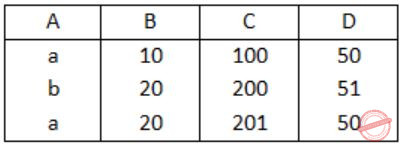Table r1: ΠA,B,C(R)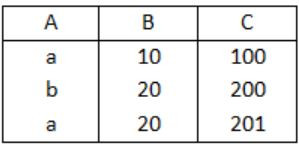Table r2: ΠA,D(R)S = r1 * r2 (* denotes natural join)
Table S:Table r ⊂ Table S
⇒ r ⊂ S
 Question 31

Consider the following C-program:

```void foo(int n, int sum) {
int k = 0, j = 0;
if (n == 0) return;
k = n % 10; j = n / 10;
sum = sum + k;
foo(j, sum);
printf ("%d,", k);
}

int main () {
int a = 2048, sum = 0;
foo(a, sum);
printf ("%d\n", sum);
}```

What does the above program print?

 A 8, 4, 0, 2, 14 B 8, 4, 0, 2, 0 C 2, 0, 4, 8, 14 D 2, 0, 4, 8, 0
Programming       C-Programming
Question 31 Explanation:
Int a=2048, Sum=0
⇒ foo (a, sum) = foo (2048,0)
⇒ n == 2048
⇒ k = n%10 = 2048%10 = 8
⇒ j = n/10 = 2048/10 = 204
Sum = Sum+k = 0+8 = 8
foo(j, sum) = foo(204, 8)
⇒ n=204
k = n%10 = 204%10 = 4
j = n/10 = 204/10 = 20
sum = sum+k = 12+0 = 12
foo(j, sum) =foo(2,12)
⇒ n=2
k = n%10 = 2%10 = 2
j = n/10 = 2/10 = 0
sum = 14
foo(0,14) ⇒ n==0
printf("%d", k) ⇒ k = 2, 0, 4, 8
After foo( ) statement one more printf statement is there then if print 0 after all digits of n.
2, 0, 4, 8, 0.
 Question 32

Consider the following C-program:

```double foo (double);    /* Line 1 */

int main() {
double da, db;

// input da

db = foo(da);
}

double foo(double a) {
return a;
} ```

The above code compiled without any error or warning. If Line 1 is deleted, the above code will show:

 A no compile warning or error B some compiler-warnings not leading to unintended results C some compiler-warnings due to type-mismatch eventually leading to unintended results D compiler errors
Programming       C-Programming
Question 32 Explanation:
When a function is called before its declaration then it leads to compiler error.
 Question 33

Postorder traversal of a given binary search tree T produces the following sequence of keys

`   10, 9, 23, 22, 27, 25, 15, 50, 95, 60, 40, 29 `

Which one of the following sequences of keys can be the result of an inorder traversal of the tree T?

 A 9, 10, 15, 22, 23, 25, 27, 29, 40, 50, 60, 95 B 9, 10, 15, 22, 40, 50, 60, 95, 23, 25, 27, 29 C 29, 15, 9, 10, 25, 22, 23, 27, 40, 60, 50, 95 D 95, 50, 60, 40, 27, 23, 22, 25, 10, 9, 15, 29
Data-Structures       Binary-Search-Tree
Question 33 Explanation:
Inorder traversal of any binary search tree is the sorted sequence of element in ascending order.
 Question 34

A Priority-Queue is implemented as a Max-Heap. Initially, it has 5 elements. The level-order traversal of the heap is given below:

`   10, 8, 5, 3, 2 `

Two new elements ”1‘ and ”7‘ are inserted in the heap in that order. The level-order traversal of the heap after the insertion of the elements is:

 A 10, 8, 7, 5, 3, 2, 1 B 10, 8, 7, 2, 3, 1, 5 C 10, 8, 7, 1, 2, 3, 5 D 10, 8, 7, 3, 2, 1, 5
Data-Structures       Queues-And-Heap
Question 34 Explanation:
Initial heap sequence: 10, 8, 5, 3, 2Insert → 1 into heap structure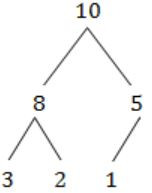Insert → 7 into heap structureHere, violating Max-heap property. So perform heapify operation.The final sequence is 10, 8, 7, 3, 2, 1, 5.
 Question 35

How many distinct binary search trees can be created out of 4 distinct keys?

 A 5 B 14 C 24 D 42
Data-Structures       Binary-Search-Tree
Question 35 Explanation:
There are 2nCn / (n+1) unlabeled trees are possible.
(or)t(0)=1
t(1)=1
t(4) = t(0)t(3) + t(1)t(2) + t(2)t(1) + t(3)t(0)
= 5+2+2+5
= 14
(or)
8C4 / 5 = 14
 Question 36

In a complete k-ary tree, every internal node has exactly k children. The number of leaves in such a tree with n internal nodes is:

 A nk B (n - 1) k + 1 C n (k - 1) + 1 D n (k - 1)
Data-Structures       K array Tree
Question 36 Explanation:
Total nodes = nk+1 (1 for root)
Leaves = total nodes - internal nodes
= nk+1-n
= n(k-1)+1
 Question 37

Suppose T(n) = 2T (n/2) + n, T(0) = T(1) = 1

Which one of the following is FALSE?

 A T(n) = O(n2) B T(n) = θ(n log n) C T(n) = Ω(n2) D T(n) = O(n log n)
Algorithms        Time-Complexity
Question 37 Explanation:
Apply masters theorem to the above example,
a=2, b=2, k=1, p=0.
The recurrence relation result will be O(n logn).
 Question 38

Let G(V,E) be an undirected graph with positive edge weights. Dijkstra's single source shortest path algorithm can be implemented using the binary heap data structure with time complexity:

 A O(|V|2) B O(|E|+|V|log|V|) C O(|V|log|V|) D O((|E|+|V|)log|V|)
Algorithms        Shortest-Path
Question 38 Explanation:
O(ElogV) equals to O((|E|+|V|)log|V|)
In worst case graph will be a complete graph i.e total edges = v(v-1)/2 where v is no. of vertices.
E<=v2
Time Complexity of Dijkstra's algorithms is:
1. With Adjacency List and Priority queue: O((v+e) log v) ⇒ O(e log v)
2. With matrix and Priority queue: O(v2 + e log v)
3. With Fibonacci Heap and adjacency list : O(e + v log v)
 Question 39

Suppose there are ⌈log n⌉ sorted lists of ⌊n/log n⌋ elements each. The time complexity of producing a sorted list of all these elements is: (Hint: Use a heap data structure)

 A O(nlog log n) B θ(nlog n) C Ω(nlog n) D Ω(n3/2)
Algorithms        Time-Complexity
Question 39 Explanation:
We can merge P sorted arrays of each size Q in O(PQ LogP)
P = log n
Q = n/log n
∴ Putting values we get,
O(n log log n)
 Question 40

Let P, Q and R be three atomic prepositional assertions. Let X denote (P ∨ Q) → R and Y denote (P → R) ∨ (Q → R). Which one of the following is a tautology?

 A X ≡ Y B X → Y C Y → X D ¬Y → X
Engineering-Mathematics       Propositional-Logic
Question 40 Explanation:
X: (P∨Q) → R
⇒ ∼(P∨Q) ∨ R
⇒ (∼P∧∼Q) ∨ R
⇒ (∼P∨R) × (∼Q∨R)
⇒ (P→R) ∧ (Q→R)
Option B: X→Y
[(P→R) × (Q→R)] → [(P→R) ∨ (Q→R)]
∼[(P→R) × (Q→R) ∨ (P→R) ∨ (Q→R)]
[∼(P→R) ∨ ∼(Q→R)] ∨ [(P→R) ∨ (Q→R)]
[∼(P→R) ∨ (P→R)] ∨ [∼(P→R) ∨ (Q→R)] ∨ [(Q→R) ∨ (P→R)] ∨ [∼(Q→R) ∨ (Q→R)]
T ∨ [∼(P→R) ∨ (Q→R)] ∨ [(Q→R) ∨ (P→R)] V T
T (✔️)
 Question 41

What is the first order predicate calculus statement equivalent to the following?

`   Every teacher is liked by some student  `
 A ∀(x) [teacher(x) → ∃ (y) [student(y) → likes (y, x)]] B ∀(x) [teacher(x) → ∃ (y) [student(y) ∧ likes (y, x)]] C ∃(y) ∀(x) [teacher(x) → [student(y) ∧ likes (y, x)]] D ∀(x) [teacher(x) ∧ ∃ (y)[student(y) → likes (y, x)]]
Engineering-Mathematics       Propositional-Logic
Question 41 Explanation:
Option A: If x is a teacher, then there exist a y such that if y is a student , then y likes x.
Option B: If x is a teacher, then there exists some y, who is a student and like x. (✔️)
Option C: There exists a student who likes all teachers.
Option D: If x is a teacher and then there exists some y, if y is a student then y likes x.
 Question 42

Let R and S be any two equivalence relations on a non-empty set A. Which one of the following statements is TRUE?

 A R∪S, R∩S are both equivalence relations. B R∪S is an equivalence relation. C R∩S is an equivalence relation. D Neither R∪S nor R∩S is an equivalence relation.
Engineering-Mathematics       Sets-And-Relation
Question 42 Explanation:
R∪S might not be transitive.
Let (a,b) present in R and (b,c) present in S and (a,c) is not present in either of them. Then R∪S will contain (a,b) and (b,c) but not (a,c) and hence not transitive.
And equivalence relation must satisfy 3 property:
(i) Reflexive
(ii) Symmetric
(iii) Transitive
But as we have seen that for R∪S, Transitivity is not satisfied.
 Question 43

Let f: B → C and g: A → B be two functions and let h = f∘g. Given that h is an onto function. Which one of the following is TRUE?

 A f and g should both be onto functions B f should be onto but g need not be onto C g should be onto but f need not be onto D both f and g need not be onto
Engineering-Mathematics       Sets And Functions
Question 43 Explanation:
Given:
f: B→C and g: A→B are two functions.
h = f∘g = f(g(x))
→ If his onto function, that means for every value in C, there must be value in A.
→ Here, we are mapping C to A using B, that means for every value in C there is a value in B then f is onto function.
→ But g may (or) may not be the onto function i.e., so values in B which may doesn't map with A.
 Question 44

What is the minimum number of ordered pairs of non-negative numbers that should be chosen to ensure that there are two pairs (a,b) and (c,d) in the chosen set such that

`    a ≡ c mod 3 and b ≡ d mod 5  `
 A 4 B 6 C 16 D 24
Engineering-Mathematics       Sets-And-Relation
Question 44 Explanation:
a = cmod3
That means a = 0,1,2 ⇒ |3|
b = dmod5
That means b = 0,1,2,3,4 ⇒ |4|
→ Total no. of order pairs = 3 * 5 = 15
→ Ordered pair (c,d) has 1 combination.
Then total no. of combinations = 15+1 = 16
 Question 45

Consider three decision problems P1, P2 and P3. It is known that P1 is decidable and P2 is undecidable. Which one of the following is TRUE?

 A P3 is decidable if P1 is reducible to P3 B P3 is undecidable if P3 is reducible to P2 C P3 is undecidable if P2 is reducible to P3 D P3 is decidable if P3 is reducible to P2's complement
Theory-of-Computation       Decidability-and-Undecidability
Question 45 Explanation:
Option A: If P1 is reducible to P3 then P3 is atleast as hard as P1. So there is no guarantee if P3 is decidable.
Option B: If P3 is reducible to P2, then P3 cannot be harder than P2. But P2 being undecidable, this can't say P3 is undecidable.
Option C: If P2 is reducible to P3, then P3 is atleast as hard as P2. Since, P2 is undecidable. This means P3 is also undecidable.
 Question 46

Consider the set H of all 3 × 3 matrices of the type

```|a   f   e|
|0   b   d|
|0   0   c|```

where a, b, c, d, e and f are real numbers and abc ≠ 0. Under the matrix multiplication operation, the set H is

 A a group B a monoid but not a group C a semi group but not a monoid D neither a group nor a semi group
Engineering-Mathematics       Sets-And-Relation
Question 46 Explanation:
abc != 0 & Identity matrix is identity then it is non-singular and inverse is also defined.
The algebraic structure is a group because the given matrix can have inverse and the inverse is non-singular.
 Question 47

Which one of the following graphs is NOT planar?A G1 B G2 C G3 D G4
Engineering-Mathematics       Graph-Theory
Question 47 Explanation:
G2 can also be drawn aswhich is planar
G3 can also be drawn aswhich is planar
G4 can also be drawn as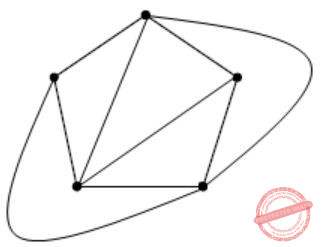which is planar
But G1 cannot be drawn as planar graph.
Hence, option (A) is the answer.
 Question 48

Consider the following system of equations in three real variables xl, x2 and x3

```2xl - x2 + 3x3 = 1
3xl- 2x2 + 5x3 = 2
-xl + 4x2 + x3 = 3 ```

This system of equations has

 A no solution B a unique solution C more than one but a finite number of solutions D an infinite number of solutions
Engineering-Mathematics       Linear-Algebra
Question 48 Explanation:2(-2 - 20) +1(3 + 5) + 3(12 - 2)
= -44 + 8 + 30
= -6 ≠ 0
→ |A| ≠ 0, we have Unique Solution.
 Question 49

What are the eigenvalues of the following 2 × 2 matrix?

```|2   -1|
|-4   5| ```
 A -1 and 1 B 1 and 6 C 2 and 5 D 4 and -1
Engineering-Mathematics       Linear-Algebra
Question 49 Explanation: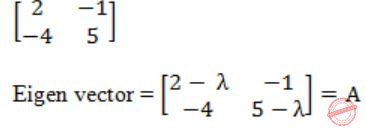|A| = (2 - λ)(5 - λ) - (4) = 0
10 - 7λ+ λ2 - 4 = 0
λ2 - 7λ + 6 = 0
λ2 - 6λ - λ + 6 = 0
(λ - 6) -1(λ - 6) = 0
λ = 1 (or) 6
 Question 50

Let, where |x|<1. What is g(i)?

 A i B i+1 C 2i D 2i
Engineering-Mathematics       Combinatorics
Question 50 Explanation:Put g(i) = i+1S = 1 + 2x + 3x2 + 4x3 + .....
Sx = 1x + 2x2 + 3x3 + 4x4 + ......
S - Sx = 1 + x + x2 + x3 + .....
[Sum of infinite series in GP with ratio < 1 is a/1-r]
S - Sx = 1/(1-x)
S(1-x) = 1/(1-x)
S = 1/(1-x)2
 Question 51

Box P has 2 red balls and 3 blue balls and box Q has 3 red balls and 1 blue ball. A ball is selected as follows:

```(i) Select a box
(ii) Choose a ball from the selected box such that each ball in
the box is equally likely to be chosen. The probabilities of
selecting boxes P and Q are (1/3) and (2/3), respectively.```

Given that a ball selected in the above process is a red ball, the probability that it came from the box P is

 A 4/19 B 5/19 C 2/9 D 19/30
Engineering-Mathematics       Probability
Question 51 Explanation:
P → 2 red, 3 blue
Q → 3 red, 1 blue
The probability of selecting a red ball is
(1/3)(2/5) + (2/3)(3/4)
2/15 + 1/2 = 19/30
The probability of selecting a red ball from P
(1/3) * (2/5) = 2/15
→ The colour of ball is selected is to be red and that is taken from the box P.
⇒ Probability of selecting a red ball from P/Probability of selecting a red ball
⇒ (2/15)/(19/30)
⇒ 4/19
 Question 52

A random bit string of length n is constructed by tossing a fair coin n times and setting a bit to 0 or 1 depending on outcomes head and tail, respectively. The probability that two such randomly generated strings are not identical is:

 A 1/2n B 1 - 1/n C 1/n! D 1-(1/2n)
Engineering-Mathematics       Probability
Question 52 Explanation:
Total combinations of strings that can be generated are 2n. We will get one such string in the first experiment. So, favourable cases for the second string are 2n - 1, so that it doesn't match with the previous generated string.
Hence Probability = (2n - 1) /2n = 1 - 1/2n
 Question 53

Consider the machine M:The language recognized by M is:

 A {w ∈ {a, b}* | every a in w is followed by exactly two b's} B {w ∈ {a, b}*| every a in w is followed by at least two b’} C {w ∈ {a, b}*| w contains the substring 'abb'} D {w ∈ {a, b}*| w does not contain 'aa' as a substring}
Theory-of-Computation       Finite-Automata
Question 53 Explanation:
Option A: It is false. The string with more than two b's also accepting the string.
Ex: abbbb, abbb...
Option B: It is True. If a string is to be accepted by given machine then it have atleast two b's.
Option C: It is false. Ex: abba. It contains 'abb' as a substring but not accepted by given machine.
Option D: It is false. Ex: abbab. It is not accepted by TM. It doesn't have 'aa' as a substring but not accepting.
 Question 54

Let Nf and Np denote the classes of languages accepted by non-deterministic finite automata and non-deterministic push-down automata, respectively. Let Df and Dp denote the classes of languages accepted by deterministic finite automata and deterministic push-down automata, respectively. Which one of the following is TRUE?

 A Df ⊂ Nf and Dp ⊂ Np B Df ⊂ Nf and Dp = Np C Df = Nf and Dp = Np D Df = Nf and Dp ⊂ Np
Theory-of-Computation       NFA
Question 54 Explanation:
NFA and DFA have equivalent powers.
So Df = Nf
NPDA can accept more languages than DPDA.
Dp ⊂ Np
 Question 55

Consider the languages:

`L1 = {anbncm |n,m > 0} and L2 = {anbmcm |n,m > 0}`

Which one of the following statements is FALSE?

 A L1 ∩ L2 is a context-free language B L1 ∪ L2 is a context-free language C L1 and L2 are context-free languages D L1 ∩ L2 is a context sensitive language
Theory-of-Computation       Context-Free-Language
Question 55 Explanation:
CFL is closed under Union.
CFL is not closed under Intersection.
L1 = {anbncm | n>0 & m>0}
L2 = {ambncn | n>0 & m>0}
L3 = L1 ∩ L2
={anbncn | n>0} It is not context-free.
 Question 56

Let L1 be a recursive language, and let L2 be a recursively enumerable but not a recursive language. Which one of the following is TRUE?

 ABCDTheory-of-Computation       Recursive-Enumerable-Languages
Question 56 Explanation:
Recursive languages are closed under complementation.
But, recursive enumerable languages are not closed under complementation.
If L1 is recursive, then L1', is also recursive.
If L2 is recursive enumerable, then L2', is not recursive enumerable language.
 Question 57

Consider the languages:

```L1 = {wwR |w ∈ {0,1}*}
L2 = {w#wR | w ∈ {0,1}*}, where # is a special symbol
L3 = {ww | w ∈ (0, 1)*}```

Which one of the following is TRUE?

 A L1 is a deterministic CFL B L2 is a deterministic CFL C L3 is a CFL, but not a deterministic CFL D L3 is a deterministic CFL
Theory-of-Computation       Context-Free-Language
Question 57 Explanation:
Given: L1 = {wwR | w ∈ {0,1}*}
→ Given L1 is CFL but not DCFL.
→ Because, we can't predict where w ends and where it reverse is starts.
→ L2 = {w#wR | w ∈ (0,1)*}
→ Given L2 is CFL and also DCFL.
→ The string w and wR are separated by special symbol '#'.
→ L3 = {ww | w ∈ (0,1)*}
This is not even a CFL. This can be proved by using pumping lemma. So, L2 is DCFL. (✔️)
 Question 58

Consider the following two problems on undirected graphs:

α: Given G(V,E), does G have an independent set of size |V|-4?
β: Given G(V,E), does G have an independent set of size 5?

Which one of the following is TRUE?

 A α is in P and β is NP-complete B α is NP-complete and β is in P C Both α and β are NP-complete D Both α and β are in P
Algorithms        P-NP
Question 58 Explanation:
Graph independent set decision problem is belongs to NP-complete.
 Question 59

Consider the grammar:

`  E → E + n | E × n | n `

For a sentence n + n × n, the handles in the right-sentential form of the reduction are:

 A n, E + n and E + n × n B n, E + n and E + E × n C n, n + n and n + n × n D n, E + n and E × n
Compiler-Design       Grammar
Question 59 Explanation:
E → E + n {Applying E → E+n}
→ E + E * n {Applying E → E * n}
→ E + n * n {Applying E → n}
→ n + n * n {Applying E → n}
We use n, E+n, E×n reductions to get a sentence n+n*n.
 Question 60

Consider the grammar:

`   S → (S) | a `

Let the number of states in SLR(1), LR(1) and LALR(1) parsers for the grammar be n1, n2 and n3 respectively. The following relationship holds good:

 A n1 < n2 < n3 B n1 = n3 < n2 C n1 = n2 = n3 D n1 ≥ n3 ≥ n2
Compiler-Design       Parsers
Question 60 Explanation:
→ SLR(1) and LALR(1) both are be the states of LR(0) items then SLR(1) = LALR(1).
→ LR(1) be the states of LR(1) items.
→ LR(0) items never be greater than LR(1) items then SLR(1) = LALR(1) < LR(1)
n1 = (n3) < (n2)
 Question 61

Consider line number 3 of the following C-program.

```int main ( ) {                   /* Line 1 */
int I, N;                      /* Line 2 */
fro (I = 0, I < N, I++);       /* Line 3 */
} ```

Identify the compiler's response about this line while creating the object-module:

 A No compilation error B Only a lexical error C Only syntactic errors D Both lexical and syntactic errors
Compiler-Design       Compilers
Question 61 Explanation:
There is no error in the above code. Actually it is a link error. Here compiler fro is a function which is not declared. Hence, it will not produce any error. It will only throw a warning in C.
 Question 62

Consider the following circuit involving a positive edge triggered D FF.Consider the following timing diagram. Let Ai represent the logic level on the line A in the i-th clock period.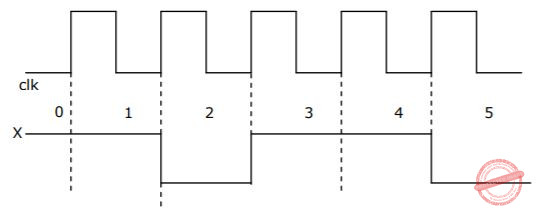Let A' represent the complement of A. The correct output sequence on Y over the clock periods 1 through 5 is

 A A0 A1 A1' A3 A4 B A0 A1 A2' A3 A4 C A1 A2 A2' A3 A4 D A1 A2' A3 A4 A5'
Digital-Logic-Design       Circuits-Output
Question 62 Explanation:Input will be accepted by the flipflop after the cycle gets finished, because +ve edge is occurring at the end of the clock cycle only.
 Question 63

The following diagram represents a finite state machine which takes as input a binary number from the least significant bit.Which one of the following is TRUE?

 A It computes 1's complement of the input number B It computes 2's complement of the input number C It increments the input number D It decrements the input number
Theory-of-Computation       Finite-Automata
Question 63 Explanation:
Let consider an example string:
Input = 1000
Output = 1111
The FSM, doesn't change until the first 1 come
I/p = 1000
1's complement = 0111
2's complement = 0111
---------------------------
1000 = I/p
----------------------------
It results 2's complement.
 Question 64

Consider the following circuit.The flip-flops are positive edge triggered D FFs. Each state is designated as a two bit string Q0Q1. Let the initial state be 00. The state transition sequence is:

 ABCDDigital-Logic-Design       Sequential-Circuits
Question 64 Explanation:Question 65

Consider a three word machine instruction

`ADD A[R0], @B`

The first operand (destination) "A[R0]" uses indexed addressing mode with R0 as the index register. The second operand (source) "@B" uses indirect addressing mode. A and B are memory addresses residing at the second and the third words, respectively. The first word of the instruction specifies the opcode, the index register designation and the source and destination addressing modes. During execution of ADD instruction, the two operands are added and stored in the destination (first operand).

The number of memory cycles needed during the execution cycle of the instruction is

 A 3 B 4 C 5 D 6
Computer-Organization       Machine-Instructions
Question 65 Explanation:
Total 4 memory cycles are required.
1 → To get 1st operand from memory address (Read).
1 → To get 2nd operand Address (Read).
1 → To get 2nd operand from the address given by previous memory (Read).
1 → To store first operand (Write).
3R + 1W = 4
 Question 66

Match each of the high level language statements given on the left hand side with the most natural addressing mode from those listed on the right hand side.

```     (1) A = B[J];     (a) Indirect addressing
(2) while [*A++];    (b) Indexed addressing
(3) int temp = *x;   (c) Auto increment```
 A (1, c), (2, b), (3, a) B (1, a), (2, c), (3, b) C (1, b), (2, c), (3, a) D (1, a), (2, b), (3, c)
Computer-Organization       Match-the-Following
Question 66 Explanation:
(a) A[i] = B[j]; Indexed, Addressing.
Here using the Index.
(b) while [*A++]; Auto increment.
The memory is increments automatically.
(c) int temp = *x; Indirect Addressing.
Here temp is assigned to integer type of Address contained in x.
 Question 67

Consider a direct mapped cache of size 32 KB with block size 32 bytes. The CPU generates 32 bit addresses. The number of bits needed for cache indexing and the number of tag bits are respectively.

 A 10, 17 B 10, 22 C 15, 17 D 5, 17
Computer-Organization       Cache
Question 67 Explanation:
No. of blocks = cache size/block size = 32KB/32 = 210
So indexing requires 10 bits.
No. of offset bit required to access 32 byte block = 5
So, No. of TAG bits = 32 - 10 - 5 = 17
 Question 68

A 5 stage pipelined CPU has the following sequence of stages:

```IF — Instruction fetch from instruction memory.
RD — Instruction decode and register read.
EX — Execute: ALU operation for data and address computation.
MA — Data memory access - for write access, the register read at RD stage is used.
WB — Register write back. ```

Consider the following sequence of instructions:

```I1 : L R0, 1oc1; R0 <= M[1oc1]
I2 : A R0, R0 1; R0 <= R0 + R0
I3 : S R2, R0 1; R2 <= R2 - R0
Let each stage take one clock cycle.```

What is the number of clock cycles taken to complete the above sequence of instructions starting from the fetch of I1?

 A 8 B 10 C 12 D 15
Computer-Organization       Pipelining
Question 68 Explanation:
From memory stage we are using operator forwarding:If we don't use operator forwarding:Total clock cycles = 8/11
There is no '11' in option.
Then no. of cycles = 8
 Question 69

A device with data transfer rate 10 KB/sec is connected to a CPU. Data is transferred byte-wise. Let the interrupt overhead be 4 μsec. The byte transfer time between the device interfaces register and CPU or memory is negligible. What is the minimum performance gain of operating the device under interrupt mode over operating it under program-controlled mode?

 A 15 B 25 C 35 D 45
Computer-Organization       Interrupt
Question 69 Explanation:
Data transfer rate = 10 KB/sec
In 1 sec it transfer 10 KB of data = 10 × 103 B = 104B
Data interrupt overhead = 4μsec = 4×10-6 sec
Transferring 10KB overhead = 4×10-6×104sec
Performance gain = 1/(4×10-6×104) = 102/4 = 25
 Question 70

Consider a disk drive with the following specifications:

16 surfaces, 512 tracks/surface, 512 sectors/track, 1 KB/sector, rotation speed 3000 rpm. The disk is operated in cycle stealing mode whereby whenever one byte word is ready it is sent to memory; similarly, for writing, the disk interface reads a 4 byte word from the memory in each DMA cycle. Memory cycle time is 40 nsec. The maximum percentage of time that the CPU gets blocked during DMA operation is:

 A 10 B 25 C 40 D 50
Computer-Organization       Secondary-Storage
Question 70 Explanation:
Time for one rotation = 60/3000
It reads 512 * 1024 B in one rotation.
Time taken to read 4B = 153ns
(153) for 4 is approximately = 160
Percentage CPU get blocked = 40*100/160 = 25
 Question 71

Suppose n processes, P1, …., Pn share m identical resource units, which can be reserved and released one at a time. The maximum resource requirement of process Pi is si, where si>0. Which one of the following is a sufficient condition for ensuring that deadlock does not occur?

 AB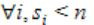CDOperating-Systems       Deadlock
Question 71 Explanation:
In the extreme situation, we have si - 1 resources and we require one more resource.
If the deadlock will never occur in the corresponding process then the following condition be true.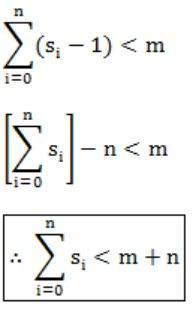Question 72

Consider the following code fragment:

```  if (fork() == 0)
{ a = a + 5; printf(“%d, %d\n”, a, &a); }
else { a = a – 5; printf(“%d, %d\n”, a, &a); }```

Let u, v be the values printed by the parent process, and x, y be the values printed by the child process. Which one of the following is TRUE?

 A u = x + 10 and v = y B u = x + 10 and v is ≠ y C u + 10 = x and v = y D u + 10 = x and v ≠ y
Operating-Systems       System-Calls
Question 72 Explanation:
u, v values printed by parent process.
u = a - 5; v be address of a
a = u + 5;
x, y values printed by child process.
x = a + 5; y be the address of a
x = u + 5 + 5; v = y
x = u + 10
 Question 73

In a packet switching network, packets are routed from source to destination along a single path having two intermediate nodes. If the message size is 24 bytes and each packet contains a header of 3 bytes, then the optimum packet size is:

 A 4 B 6 C 7 D 9
Computer-Networks       Network-Switching
Question 73 Explanation:Packet size have minimum header overhead.
 Question 74

Suppose the round trip propagation delay for a 10 Mbps Ethernet having 48-bit jamming signal is 46.4 μs. The minimum frame size is:

 A 94 B 416 C 464 D 512
Computer-Networks       Ethernet
Question 74 Explanation:
Given RTT = 46.4 μs, B.w. = 10 Mbps
Round trip propagation delay is RTT = 2*Tp
Minimum frame size of Ethernet can be found by using formula Tt = 2*Tp
Let L is minimum frame size. Then L / 10Mbps = 46.4 μs
L = 464 Kbits
It has nothing to do with jamming signal.
 Question 75

Let E1 and E2 be two entities in an E/R diagram with simple single-valued attributes. R1 and R2 are two relationships between E1 and E2, where R1 is one-to-many and R2 is many-to-many. R1 and R2 do not have any attributes of their own. What is the minimum number of tables required to represent this situation in the relational model?

 A 2 B 3 C 4 D 5
Computer-Networks       ER-Model
Question 75 Explanation:
R1 and R2 two relationships between E1 and E2.
R1 is one to many.
R2 is many to many.
→ E1 and E2 have separate table because they need to store multiple values.
→ R2 also have separate table by considering Primary keys E1 and E2 as foreign keys.
→ R1 is converted to many side table i.e., E2 as Primary key and E1 as Foreign key.
So, totally we need 3 tables to store the value.
 Question 76

The following table has two attributes A and C where A is the primary key and C is the foreign key referencing A with on-delete cascade.

```          A   C
-----
2   4
3   4
4   3
5   2
7   2
9   5
6   4```

The set of all tuples that must be additionally deleted to preserve referential integrity when the tuple (2,4) is deleted is:

 A (3,4) and (6,4) B (5,2) and (7,2) C (5,2), (7,2) and (9,5) D (3,4), (4,3) and (6,4)
Database-Management-System       Referential-Integrity
Question 76 Explanation:
If (2,4) is deleted and that results foreign key of value with 2 also deleted i.e., (5,2), (7,2) are also delete. And again deleting Primary keys (5), (7) means delete the foreign key value (5,7) that results (9,5) also deleted.
Totally (5,2), (7,2), (9,5) are also deleted.
 Question 77
The relation book (title, price) contains the titles and prices of different books. Assuming that no two books have the same price, what does the following SQL query list?
```     select title
from book as B
where (select count(*)
from book as T
where T.price > B.price) < 5 ```
 A Titles of the four most expensive books B Title of the fifth most inexpensive book C Title of the fifth most expensive book D Titles of the five most expensive books
Database-Management-System       SQL
Question 77 Explanation:
Which results titles of the five most expensive books.
The where clause of outer query will be true for 5 most expensive books.
 Question 78

Consider a relation scheme R = (A, B, C, D, E, H) on which the following functional dependencies hold: {A → B, BC → D, E → C, D → A}. What are the candidate keys of R?

 A AE, BE B AE, BE, DE C AEH, BEH, BCH D AEH, BEH, DEH
Database-Management-System       Functional-Dependency
Question 78 Explanation:
Using the given functional dependencies and looking at the dependent attributes, E and H are not dependent on any. So, they must be a part of any candidate key.
So only option (D) contains E and H as part of candidate key.
 Question 79

Consider the following data path of a CPU.The, ALU, the bus and all the registers in the data path are of identical size. All operations including incrementation of the PC and the GPRs are to be carried out in the ALU. Two clock cycles are needed for memory read operation - the first one for loading address in the MAR and the next one for loading data from the memory bus into the MDR.

The instruction "add R0, R1" has the register transfer interpretation R0 <= R0+R1. The minimum number of clock cycles needed for execution cycle of this instruction is:

 A 2 B 3 C 4 D 5
Computer-Organization       Machine-Instructions
Question 79 Explanation:
1 cycle → Increment
1 cycle → Load PC to MAR
1 cycle → Fetch memory and load to PC
----------------------------------------------
3 cycles
----------------------------------------------
 Question 80

Consider the following data path of a CPU.The, ALU, the bus and all the registers in the data path are of identical size. All operations including incrementation of the PC and the GPRs are to be carried out in the ALU. Two clock cycles are needed for memory read operation - the first one for loading address in the MAR and the next one for loading data from the memory bus into the MDR.

The instruction "call Rn, sub" is a two word instruction. Assuming that PC is incremented during the fetch cycle of the first word of the instruction, its register transfer interpretation is

```      Rn <= PC + 1;
PC <= M[PC]; ```

The minimum number of CPU clock cycles needed during the execution cycle of this instruction is:

 A 2 B 3 C 4 D 5
Computer-Organization       Machine-Instructions
Question 80 Explanation:
1 cycle → Increment
1 cycle → Load PC to MAR
1 cycle → Fetch memory and load to PC
----------------------------------------------
3 cycles
----------------------------------------------
 Question 81

Consider the following C-function:

```double foo (int n) {
int i;
double sum;
if (n == 0) return 1.0;
else {
sum = 0.0;
for (i = 0; i < n; i++)
sum += foo(i);
return sum;
}  }   ```

The space complexity of the above function is:

 A O(1) B O(n) C O(n!) D O(nn)
Algorithms        Space-complexity
Question 81 Explanation:
The code here is storing only local variables. So, the space complexity will be the recursion depth- maximum happening for the last iteration of the for loop- foo(n-1) - foo(n-2) - .... - foo(0) all giving space complexity O(n).
Time complexity is O(2n).
foo(n) = foo(n-1) + foo(n-2) + ... + 1
foo(n-1) = foo(n-2) + foo(n-3) + ...
=> foo(n) = 2*foo(n-1), foo(0) = 1
=> foo(n) is in O(2n) [By recursion tree method you can solve it].
 Question 82

Consider the following C-function:

```double foo (int n) {
int i;
double sum;
if (n == 0) return 1.0;
else {
sum = 0.0;
for (i = 0; i < n; i++)
sum += foo(i);
return sum;
}  }   ```

Suppose we modify the above function foo() and store the values of foo (i), 0 < = i < n, as and when they are computed. With this modification, the time complexity for function foo() is significantly reduced. The space complexity of the modified function would be:

 A O(1) B O(n) C O(n2) D O(n!)
Algorithms        Space-complexity
Question 82 Explanation:
To store the n values we need space complexity O(n). So, the space complexity won't change and will be O(n).
 Question 83

Let s and t be two vertices in a undirected graph G=(V,E) having distinct positive edge weights. Let [X, Y] be a partition of V such that s ∈ X and t ∈ Y. Consider the edge e having the minimum weight amongst all those edges that have one vertex in X and one vertex in Y.

The edge e must definitely belong to:

 A the minimum weighted spanning tree of G B the weighted shortest path from s to t C each path from s to t D the weighted longest path from s to t
Data-Structures       Graphs
Question 83 Explanation:
Since every edge has distinct edge weights. So, the edge with minimum weight will definitely be present in MST.
 Question 84

Let s and t be two vertices in a undirected graph G=(V,E) having distinct positive edge weights. Let [X, Y] be a partition of V such that s ∈ X and t ∈ Y. Consider the edge e having the minimum weight amongst all those edges that have one vertex in X and one vertex in Y.

Let the weight of an edge e denote the congestion on that edge. The congestion on a path is defined to be the maximum of the congestions on the edges of the path. We wish to find the path from s to t having minimum congestion. Which one of the following paths is always such a path of minimum congestion?

 A a path from s to t in the minimum weighted spanning tree B a weighted shortest path from s to t C an Euler walk from s to t D a Hamiltonian path from s to t
Data-Structures       Graphs
Question 84 Explanation:
Let us first understand what is minimum congestion actually.
Minimum congestion is the edge with maximum weight among all other edges included in the path.
Now suppose shortest path from A→B is 6, but in MST, we have A→C→B(A→C=4, C→B=3), then along the path in MST, we have minimum congestion i.e., 4.
 Question 85

Consider the following expression grammar. The seman­tic rules for expression calculation are stated next to each grammar production.

```E → number 	 E.val = number. val
|E '+' E 	 E(1).val = E(2).val + E>sup>(3).val
|E '×' E	 E(1).val = E(2).val × E(3).val```

The above grammar and the semantic rules are fed to a yacc tool (which is an LALR(1) parser generator) for parsing and evaluating arithmetic expressions. Which one of the following is true about the action of yacc for the given grammar?

 A It detects recursion and eliminates recursion B It detects reduce-reduce conflict, and resolves C It detects shift-reduce conflict, and resolves the conflict in favor of a shift over a reduce action D It detects shift-reduce conflict, and resolves the conflict in favor of a reduce over a shift action
Compiler-Design       Parsres
Question 85 Explanation:
Yacc favours shift move in case of SR conflict.
 Question 86

Consider the following expression grammar. The seman­tic rules for expression calculation are stated next to each grammar production.

```E → number 	 E.val = number. val
|E '+' E 	 E(1).val = E(2).val + E>sup>(3).val
|E '×' E	 E(1).val = E(2).val × E(3).val```

Assume the conflicts in Part (a) of this question are resolved and an LALR(1) parser is generated for parsing arithmetic expressions as per the given grammar. Consider an expression 3 × 2 + 1. What precedence and associativity properties does the generated parser realize?

 A Equal precedence and left associativity; expression is evaluated to 7 B Equal precedence and right associativity; expression is evaluated to 9 C Precedence of '×' is higher than that of '+', and both operators are left associative; expression is evaluated to 7 D Precedence of '+' is higher than that of '×', and both operators are left associative; expression is evaluated to 9
Compiler-Design       Parsers
Question 86 Explanation:
First of all, it is ambiguous grammar. Hence, equal precedence and associativity. Now as Yacc resolved it with shift move we will shift until the last operator and then we will start reducing.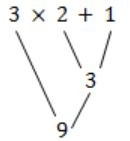Hence, the answer is 9 and right associative.
 Question 87

We are given 9 tasks T1, T2.... T9. The execution of each task requires one unit of time. We can execute one task at a time. Each task Ti has a profit Pi and a deadline di Profit Pi is earned if the task is completed before the end of the dith unit of time.

```Task     T1  T2	 T3  T4  T5  T6	 T7 T8  T9
Profit   15  20	 30  18  18  10	 23 16  25
Deadline 7   2 	 5   3 	 4   5 	 2  7   3```

Are all tasks completed in the schedule that gives maximum profit?

 A All tasks are completed B T1 and T6 are left out C T1 and T8 are left out D T4 and 6 are left out
Algorithms        Greedy-Algorithm
Question 87 Explanation:
First sort the tasks in descending order of their profit.
T3 T9 T7 T2 T4 T5 T8 T1 T6
Now the maximum deadline given is 7.
So we will take Array of 7 elements. And will try to put the tasks starting from T3 to T6, upto their maximum deadline possible.So T4 and T6 are left out.
 Question 88

We are given 9 tasks T1, T2.... T9. The execution of each task requires one unit of time. We can execute one task at a time. Each task Ti has a profit Pi and a deadline di Profit Pi is earned if the task is completed before the end of the dith unit of time.

```Task     T1  T2	 T3  T4  T5  T6	 T7 T8  T9
Profit   15  20	 30  18  18  10	 23 16  25
Deadline 7   2 	 5   3 	 4   5 	 2  7   3```

What is the maximum profit earned?

 A 147 B 165 C 167 D 175
Algorithm       Greedy-Algorithm
Question 88 Explanation:
We will now add the profits of the tasks we have put in the array.
30+25+23+20+18+16+15 = 147
 Question 89

Consider the following floating point format.Mantissa is a pure fraction in sign-magnitude form.

The decimal number 0.239 × 213 has the following hexadecimal representation (without normalization and rounding off:

 A 0D 24 B 0D 4D C 4D 0D D 4D 3D
Digital-Logic-Design       Number-Systems
Question 89 Explanation:
Sign Bit = 0
Convert 0.239 to binary
0.239 * 2 = 0.478
0.478 * 2 = 0.956
0.956 * 2 = 1.912
0.912 * 2 = 1.824
0.824 * 2 = 1.648
0.648 * 2 = 1.296
0.296 * 2 = 0.512
0.512 * 2 = 1.024
Mantissa = (0. 00111101)2
Bias = 64. So biased exponent is 13+64 = 77= (1001101)2
0.239 × 213 = 0 1001101 00111101
= 0100 1101 0011 1101
= 4 D 3 D
 Question 90

Consider the following floating point format.Mantissa is a pure fraction in sign-magnitude form.

The normalized representation for the above format is specified as follows. The mantissa has an implicit 1 preceding the binary (radix) point. Assume that only 0's are padded in while shifting a field. The normalized representation of the above number (0.239 × 213) is

 A 0A 20 B 11 34 C 4D D0 D 4A E8
Digital-Logic-Design       Number-Systems
Question 90 Explanation:
Sign Bit = 0
Convert 0.239 to binary
0.239 * 2 = 0.478
0.478 * 2 = 0.956
0.956 * 2 = 1.912
0.912 * 2 = 1.824
0.824 * 2 = 1.648
0.648 * 2 = 1.296
0.296 * 2 = 0.512
0.512 * 2 = 1.024
Mantissa = (0. 00111101)2
0.239 × 213 = 1.11101000 x 210 <- Normalized Mantissa
Bias = 64. So biased exponent is 10+64 = 74 = (1001010)2
0.239 × 213 = 0 1001010 11101000
= 0100 1010 1110 1000
= (4 A E 8)16
There are 90 questions to complete.
PHP Code Snippets Powered By : XYZScripts.com
error: Content is protected !!